## 4.3 Making a Line Graph with Multiple Lines

### 4.3.1 Problem

You want to make a line graph with more than one line.

### 4.3.2 Solution

In addition to the variables mapped to the x- and y-axes, map another (discrete) variable to colour or linetype, as shown in Figure 4.6:

``````library(gcookbook) # Load gcookbook for the tg data set

# Map supp to colour
ggplot(tg, aes(x = dose, y = length, colour = supp)) +
geom_line()

# Map supp to linetype
ggplot(tg, aes(x = dose, y = length, linetype = supp)) +
geom_line()``````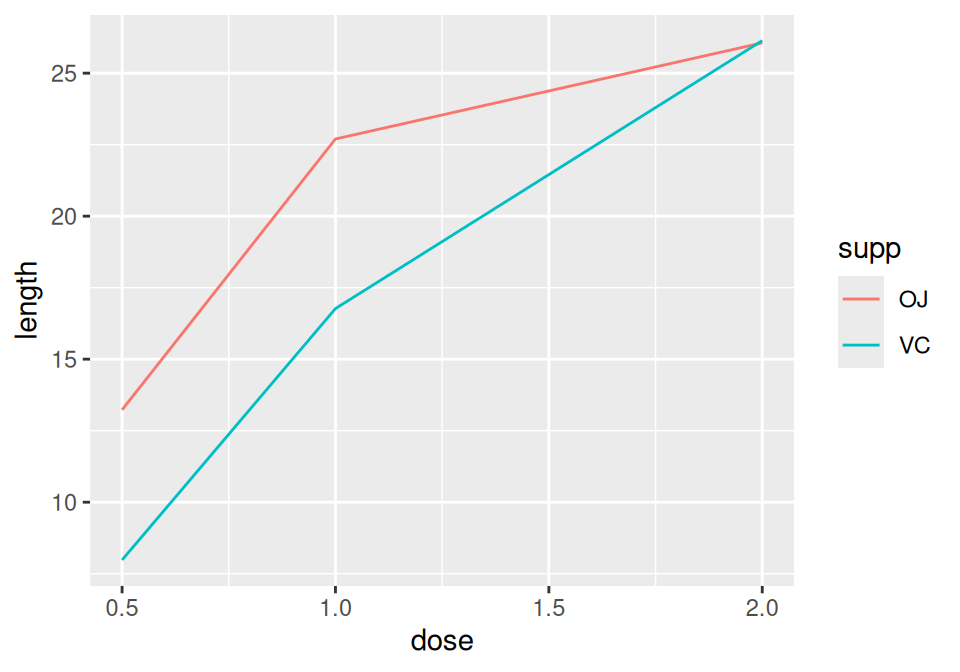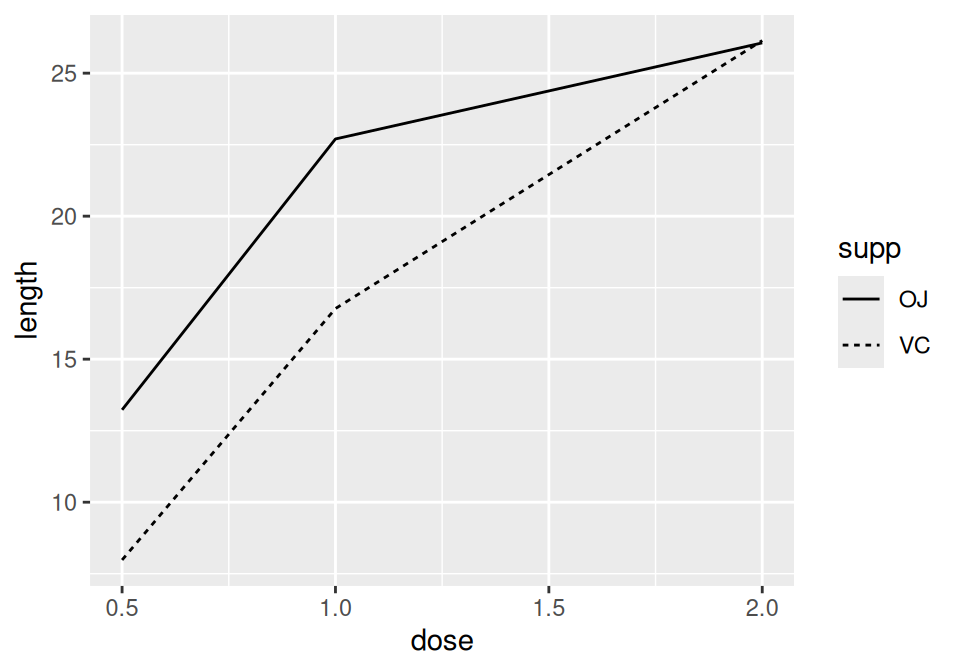Figure 4.6: A variable mapped to colour (left); A variable mapped to linetype (right)

### 4.3.3 Discussion

The `tg` data has three columns, including the factor `supp`, which we mapped to `colour` and `linetype`:

``````tg
#>   supp dose length
#> 1   OJ  0.5  13.23
#> 2   OJ  1.0  22.70
#> 3   OJ  2.0  26.06
#> 4   VC  0.5   7.98
#> 5   VC  1.0  16.77
#> 6   VC  2.0  26.14``````

Note

If the x variable is a factor, you must also tell ggplot to group by that same variable, as described below.

Line graphs can be used with a continuous or categorical variable on the x-axis. Sometimes the variable mapped to the x-axis is conceived of as being categorical, even when it’s stored as a number. In the example here, there are three values of dose: 0.5, 1.0, and 2.0. You may want to treat these as categories rather than values on a continuous scale. To do this, convert `dose` to a factor (Figure 4.7):

``````ggplot(tg, aes(x = factor(dose), y = length, colour = supp, group = supp)) +
geom_line()``````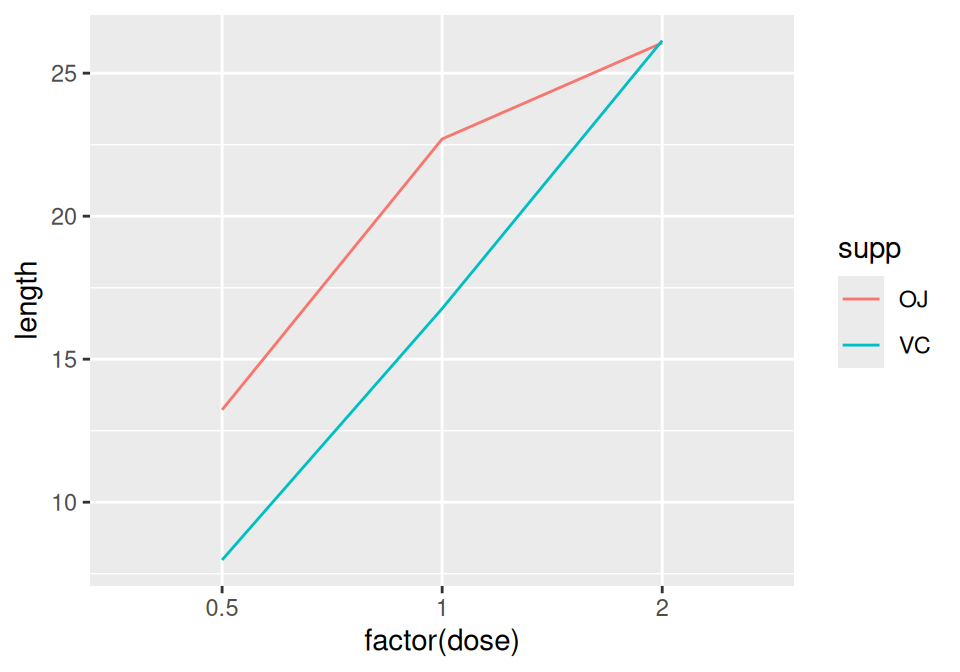Figure 4.7: Line graph with continuous x variable converted to a factor

Notice the use of `group = supp`. Without this statement, ggplot won’t know how to group the data together to draw the lines, and it will give an error:

``````ggplot(tg, aes(x = factor(dose), y = length, colour = supp)) + geom_line()
#> geom_path: Each group consists of only one observation. Do you need to

Another common problem when the incorrect grouping is used is that you will see a jagged sawtooth pattern, as in Figure 4.8:

``````ggplot(tg, aes(x = dose, y = length)) +
geom_line()``````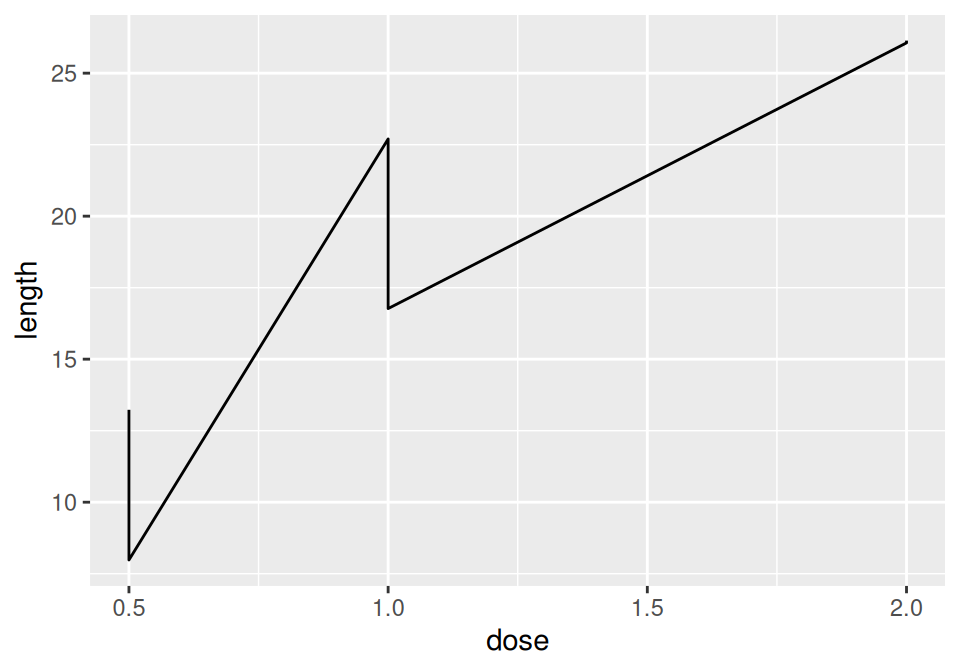Figure 4.8: A sawtooth pattern indicates improper grouping

This happens because there are multiple data points at each y location, and ggplot thinks they’re all in one group. The data points for each group are connected with a single line, leading to the sawtooth pattern. If any discrete variables are mapped to aesthetics like colour or linetype, they are automatically used as grouping variables. But if you want to use other variables for grouping (that aren’t mapped to an aesthetic), they should be used with group.

Note

When in doubt, if your line graph looks wrong, try explicitly specifying the grouping variable with group. It’s common for problems to occur with line graphs because ggplot is unsure of how the variables should be grouped.

If your plot has points along with the lines, you can also map variables to properties of the points, such as shape and fill (Figure 4.9):

``````ggplot(tg, aes(x = dose, y = length, shape = supp)) +
geom_line() +
geom_point(size = 4)  # Make the points a little larger

ggplot(tg, aes(x = dose, y = length, fill = supp)) +
geom_line() +
geom_point(size = 4, shape = 21)  # Also use a point with a color fill``````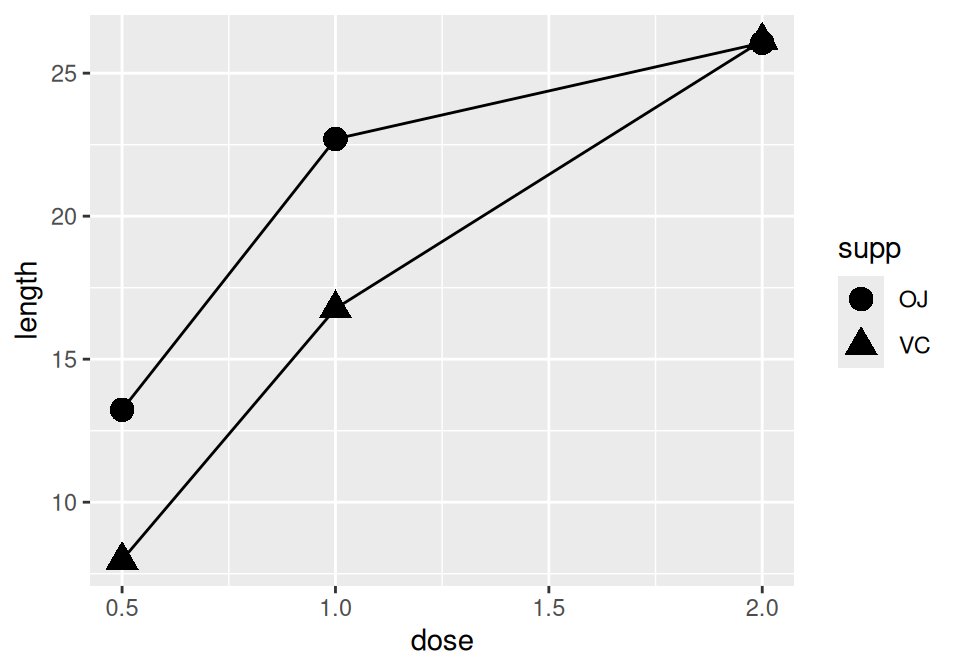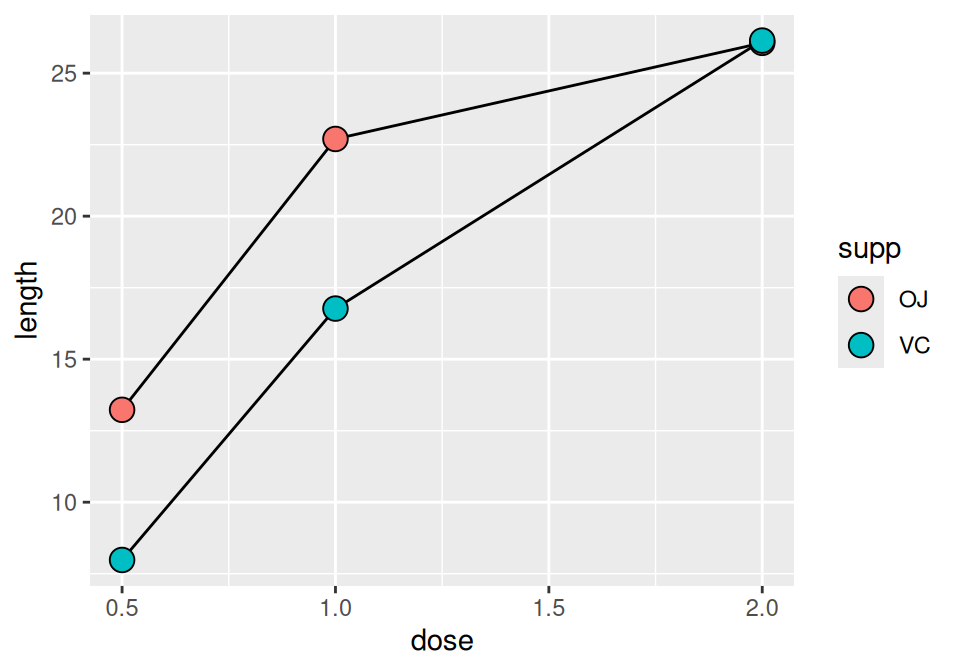Figure 4.9: Line graph with different shapes (left); With different colors (right)

Sometimes points will overlap. In these cases, you may want to dodge them, which means their positions will be adjusted left and right (Figure 4.10). When doing so, you must also dodge the lines, or else only the points will move and they will be misaligned. You must also specify how far they should move when dodged:

``````ggplot(tg, aes(x = dose, y = length, shape = supp)) +
geom_line(position = position_dodge(0.2)) +           # Dodge lines by 0.2
geom_point(position = position_dodge(0.2), size = 4)  # Dodge points by 0.2``````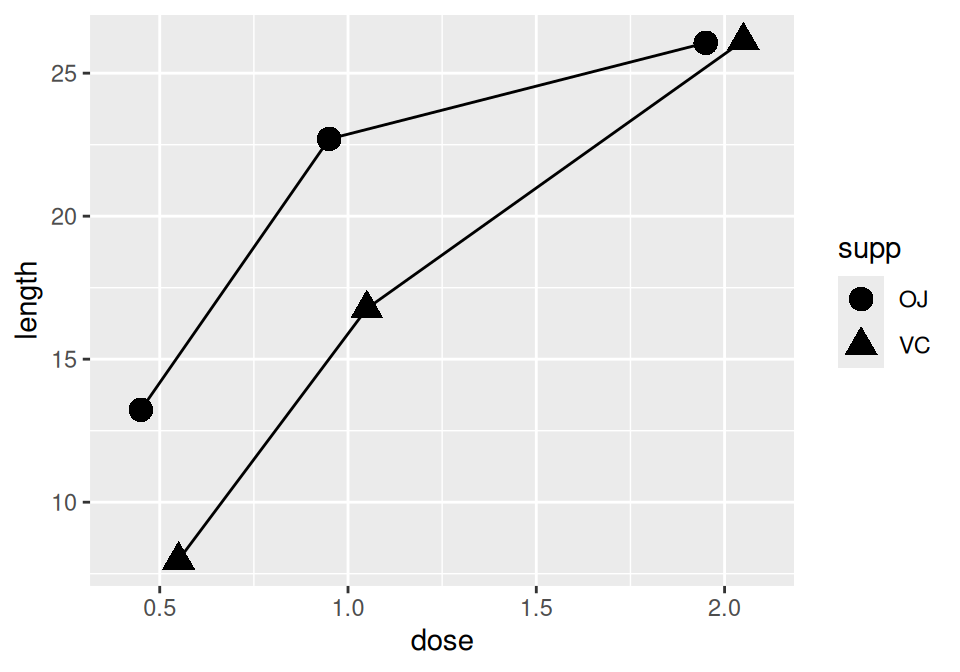Figure 4.10: Dodging to avoid overlapping points